# 5.2 Solve systems of equations by substitution  (Page 2/5)

 Page 2 / 5

Solve the system by substitution. $\left\{\begin{array}{c}3x+y=5\hfill \\ 2x+4y=-10\hfill \end{array}$

## Solution

We need to solve one equation for one variable. Then we will substitute that expression into the other equation.

 Solve for y . Substitute into the other equation.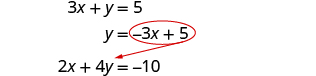Replace the y with −3 x + 5.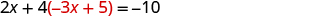Solve the resulting equation for x .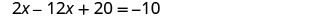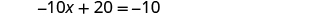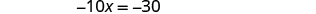Substitute x = 3 into 3 x + y = 5 to find y .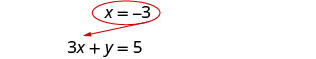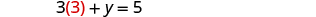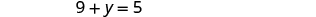The ordered pair is (3, −4).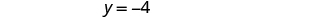Check the ordered pair in both equations: $\begin{array}{cccc}\begin{array}{ccc}\hfill 3x+y& =\hfill & 5\hfill \\ \hfill 3·3+\left(-4\right)& \stackrel{?}{=}\hfill & 5\hfill \\ \hfill 9-4& \stackrel{?}{=}\hfill & 5\hfill \\ \hfill 5& =\hfill & 5\phantom{\rule{0.2em}{0ex}}✓\hfill \end{array}& & & \begin{array}{ccc}\hfill 2x+4y& =\hfill & -10\hfill \\ \hfill 2·3+4\left(-4\right)& =\hfill & -10\hfill \\ \hfill 6-16& \stackrel{?}{=}\hfill & -10\hfill \\ \hfill -10& =\hfill & -10\phantom{\rule{0.2em}{0ex}}✓\hfill \end{array}\end{array}$ The solution is (3, −4).

Solve the system by substitution. $\left\{\begin{array}{c}4x+y=2\hfill \\ 3x+2y=-1\hfill \end{array}$

$\left(1,-2\right)$

Solve the system by substitution. $\left\{\begin{array}{c}-x+y=4\hfill \\ 4x-y=2\hfill \end{array}$

$\left(2,6\right)$

In [link] it was easiest to solve for y in the first equation because it had a coefficient of 1. In [link] it will be easier to solve for x .

Solve the system by substitution. $\left\{\begin{array}{c}x-2y=-2\hfill \\ 3x+2y=34\hfill \end{array}$

## Solution

We will solve the first equation for $x$ and then substitute the expression into the second equation.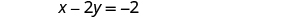Solve for x . Substitute into the other equation.Replace the x with 2 y − 2.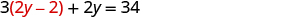Solve the resulting equation for y .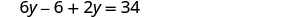Substitute y = 5 into x − 2 y = −2 to find x .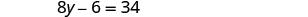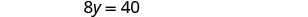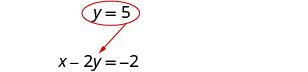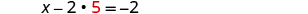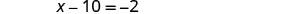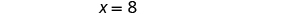The ordered pair is (8, 5). Check the ordered pair in both equations: $\begin{array}{cccc}\begin{array}{ccc}\hfill x-2y& =\hfill & -2\hfill \\ \hfill 8-2·5& \stackrel{?}{=}\hfill & -2\hfill \\ \hfill 8-10& \stackrel{?}{=}\hfill & -2\hfill \\ \hfill -2& =\hfill & -2\phantom{\rule{0.2em}{0ex}}✓\hfill \end{array}& & & \begin{array}{ccc}\hfill 3x+2y& =\hfill & 34\hfill \\ \hfill 3·8+2·5& \stackrel{?}{=}\hfill & 34\hfill \\ \hfill 24+10& \stackrel{?}{=}\hfill & 34\hfill \\ \hfill 34& =\hfill & 34\phantom{\rule{0.2em}{0ex}}✓\hfill \end{array}\end{array}$ The solution is (8, 5).

Solve the system by substitution. $\left\{\begin{array}{c}x-5y=13\hfill \\ 4x-3y=1\hfill \end{array}$

$\left(-2,-3\right)$

Solve the system by substitution. $\left\{\begin{array}{c}x-6y=-6\hfill \\ 2x-4y=4\hfill \end{array}$

$\left(6,2\right)$

When both equations are already solved for the same variable, it is easy to substitute!

Solve the system by substitution. $\left\{\begin{array}{c}y=-2x+5\hfill \\ y=\frac{1}{2}x\hfill \end{array}$

## Solution

Since both equations are solved for y , we can substitute one into the other.

 Substitute $\frac{1}{2}x$ for y in the first equation.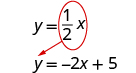Replace the y with $\frac{1}{2}x.$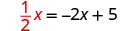Solve the resulting equation. Start by clearing the fraction.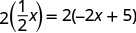Solve for x .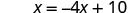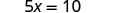Substitute x = 2 into y = $\frac{1}{2}x$ to find y .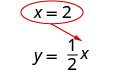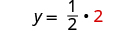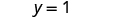The ordered pair is (2,1). Check the ordered pair in both equations: $\begin{array}{cccc}\begin{array}{ccc}\hfill y& =\hfill & \frac{1}{2}x\hfill \\ \hfill 1& \stackrel{?}{=}\hfill & \frac{1}{2}·2\hfill \\ \hfill 1& =\hfill & 1\phantom{\rule{0.2em}{0ex}}✓\hfill \end{array}& & & \begin{array}{ccc}\hfill y& =\hfill & -2x+5\hfill \\ \hfill 1& \stackrel{?}{=}\hfill & -2·2+5\hfill \\ \hfill 1& =\hfill & -4+5\hfill \\ \hfill 1& =\hfill & 1\phantom{\rule{0.2em}{0ex}}✓\hfill \end{array}\end{array}$ The solution is (2,1).

Solve the system by substitution. $\left\{\begin{array}{c}y=3x-16\hfill \\ y=\frac{1}{3}x\hfill \end{array}$

$\left(6,2\right)$

Solve the system by substitution. $\left\{\begin{array}{c}y=\text{−}x+10\hfill \\ y=\frac{1}{4}x\hfill \end{array}$

$\left(8,2\right)$

Be very careful with the signs in the next example.

Solve the system by substitution. $\left\{\begin{array}{c}4x+2y=4\hfill \\ 6x-y=8\hfill \end{array}$

## Solution

We need to solve one equation for one variable. We will solve the first equation for y .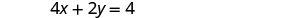Solve the first equation for y .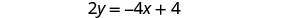Substitute −2 x + 2 for y in the second equation.Replace the y with −2 x + 2.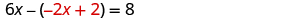Solve the equation for x .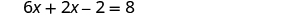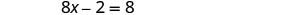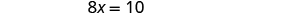Substitute $x=\frac{5}{4}$ into 4 x + 2 y = 4 to find y .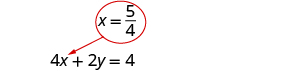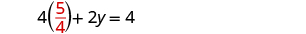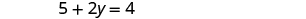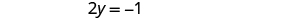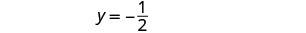The ordered pair is $\left(\frac{5}{4},-\frac{1}{2}\right).$ Check the ordered pair in both equations. $\begin{array}{cccc}\begin{array}{ccc}\hfill 4x+2y& =\hfill & 4\hfill \\ \hfill 4\left(\frac{5}{4}\right)+2\left(-\frac{1}{2}\right)& \stackrel{?}{=}\hfill & 4\hfill \\ \hfill 5-1& \stackrel{?}{=}\hfill & 4\hfill \\ \hfill 4& =\hfill & 4\phantom{\rule{0.2em}{0ex}}✓\hfill \\ \\ \\ \\ \\ \end{array}\hfill & & & \begin{array}{ccc}\hfill 6x-y& =\hfill & 8\hfill \\ \hfill 6\left(\frac{5}{4}\right)-\left(-\frac{1}{2}\right)& \stackrel{?}{=}\hfill & 8\hfill \\ \hfill \frac{15}{4}-\left(-\frac{1}{2}\right)& \stackrel{?}{=}\hfill & 8\hfill \\ \hfill \frac{16}{2}& \stackrel{?}{=}\hfill & 8\hfill \\ \hfill 8& =\hfill & 8\phantom{\rule{0.2em}{0ex}}✓\hfill \end{array}\hfill \end{array}$ The solution is $\left(\frac{5}{4},-\frac{1}{2}\right).$

Solve the system by substitution. $\left\{\begin{array}{c}x-4y=-4\hfill \\ -3x+4y=0\hfill \end{array}$

$\left(2,\frac{3}{2}\right)$

Solve the system by substitution. $\left\{\begin{array}{c}4x-y=0\hfill \\ 2x-3y=5\hfill \end{array}$

$\left(-\frac{1}{2},-2\right)$

In [link] , it will take a little more work to solve one equation for x or y .

Solve the system by substitution. $\left\{\begin{array}{c}4x-3y=6\hfill \\ 15y-20x=-30\hfill \end{array}$

## Solution

We need to solve one equation for one variable. We will solve the first equation for x .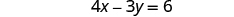Solve the first equation for x .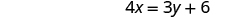Substitute $\frac{3}{4}y+\frac{3}{2}$ for x in the second equation.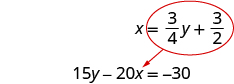Replace the x with $\frac{3}{4}y+\frac{3}{2}.$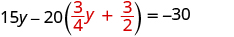Solve for y .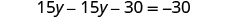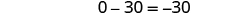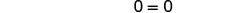Since 0 = 0 is a true statement, the system is consistent. The equations are dependent. The graphs of these two equations would give the same line. The system has infinitely many solutions.

Aziza is solving this equation-2(1+x)=4x+10
No. 3^32 -1 has exactly two divisors greater than 75 and less than 85 what is their product?
x^2+7x-19=0 has Two solutions A and B give your answer to 3 decimal places
3. When Jenna spent 10 minutes on the elliptical trainer and then did circuit training for20 minutes, her fitness app says she burned 278 calories. When she spent 20 minutes onthe elliptical trainer and 30 minutes circuit training she burned 473 calories. How manycalories does she burn for each minute on the elliptical trainer? How many calories doesshe burn for each minute of circuit training?
.473
Angelita
?
Angelita
John left his house in Irvine at 8:35 am to drive to a meeting in Los Angeles, 45 miles away. He arrived at the meeting at 9:50. At 3:30 pm, he left the meeting and drove home. He arrived home at 5:18.
p-2/3=5/6 how do I solve it with explanation pls
P=3/2
Vanarith
1/2p2-2/3p=5p/6
James
Cindy
4.5
Ruth
is y=7/5 a solution of 5y+3=10y-4
yes
James
Cindy
Lucinda has a pocketful of dimes and quarters with a value of $6.20. The number of dimes is 18 more than 3 times the number of quarters. How many dimes and how many quarters does Lucinda have? Rhonda Reply Find an equation for the line that passes through the point P ( 0 , − 4 ) and has a slope 8/9 . Gabriel Reply is that a negative 4 or positive 4? Felix y = mx + b Felix if negative -4, then -4=8/9(0) + b Felix -4=b Felix if positive 4, then 4=b Felix then plug in y=8/9x - 4 or y=8/9x+4 Felix Macario is making 12 pounds of nut mixture with macadamia nuts and almonds. macadamia nuts cost$9 per pound and almonds cost $5.25 per pound. how many pounds of macadamia nuts and how many pounds of almonds should macario use for the mixture to cost$6.50 per pound to make?
Nga and Lauren bought a chest at a flea market for $50. They re-finished it and then added a 350 % mark - up Makaila Reply$1750
Cindy
the sum of two Numbers is 19 and their difference is 15
2, 17
Jose
interesting
saw
4,2
Cindy
Felecia left her home to visit her daughter, driving 45mph. Her husband waited for the dog sitter to arrive and left home 20 minutes, or 13 hour later. He drove 55mph to catch up to Felecia. How long before he reaches her?
hola saben como aser un valor de la expresión
NAILEA
integer greater than 2 and less than 12
2 < x < 12
Felix
I'm guessing you are doing inequalities...
Felix
Actually, translating words into algebraic expressions / equations...
Felix
hi
Darianna
hello
Mister
Eric here
Eric
6
Cindy

#### Get Jobilize Job Search Mobile App in your pocket Now!By Mary CohenBy Richley CrapoBy Robert MurphyBy Yasser IbrahimBy Madison ChristianBy Janet ForresterBy Brooke DelaneyBy OpenStaxBy Jazzycazz JacksonBy Yasser Ibrahim